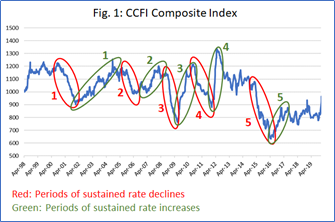home  /  login  /  register  /  search  /  Tel: 020 8844 2266

# Rate reduction could take 18-30 months

## In issue 540 of the Sunday Spotlight, we analysed the composite CCFI freight rate index, to see how quickly freight rates could normalise, if using history as a guide.In figure 1, we narrowed down the CCFI to the pre-pandemic data, and identified 5 periods of sustained rate decreases (marked in red), as well as 5 periods of sustained rate increases (marked in green). Based on this, we defined the time periods of increases and decreases, and analysed each separately. We measured the rate of decline in terms of the average percentage point drop per week, over the full period of each of the 5 decline periods. This ranged between -0.4% and -0.9% per week. If these time periods are to reflect the inherent pricing mechanisms in the industry, we can use them to calculate a reversal back to normality. This, however, presents the next question: what is a normal rate level?

Based on historical CCFI data, this is represented by rate levels around index level 1000. This represents a decline of -69% from the current rate level. During the global financial crisis of 2008-2009, rates declined at the fastest rate of a weekly decline of -0.9%, and if we apply this speed of decline to the current rate levels, it would take 18 months to get back to “normal”. If, however, the rate of decline matches the average seen over the 5 rate decline periods, then normalization would take as much as 26 months. However, it can be argued that the current increase is much stronger than before, and that should be accounted for.

To do this, we calculated the average weekly rate increases for the 5 periods with rate increases. On average, over the 5 periods of decline, the rates dropped -0.6 percentage points per week, on average. Over the 5 periods of increase, we saw rates go up by 1.1 percentage points over the period. This implies a factor of 1.8 between increases and decreases, meaning that rate increases tend to be 80% stronger, on a weekly basis, than decreases. As the current rate level comes after a 17-month period of sustained rate increases, the result becomes 30 months before a reversal back to index 1000.

Source: Sea Intelligence